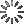• Share

# Particles Explosion with HTML5 CanvasKushagra Agarwal

Particles Explosion using `canvas`.

The basic idea here is to generate 500 circles from center having different radii, velocities and colors. Then, decrementing their radius in each consecutive frame so that it finally become zero and the circle dies. Now each died circle is then regenerated from the center so that the explosion never ends. You can see how is everything done by looking at the code fragments below.

This is how I created the circles with different properties.

```function create() {
//Place the circles at the center
this.x = W/2;
this.y = H/2;

//Random radius between 2 and 6

//Random velocities between -10 and +10 (negative value so that they move in both directions)
this.vx = -10 + Math.random()*20;
this.vy = -10 + Math.random()*20;

//Random colors
this.r = Math.round(Math.random())*255;
this.g = Math.round(Math.random())*255;
this.b = Math.round(Math.random())*255;
}

for (var i = 0; i < 500; i++) {
circles.push(new create());
}
```

In the `for` loop, a new random circle is pushed into the i-th position. So now, we have an array of circles that have different properties. Now it's time to burst them out.

```//Fill the canvas with circles
for(var j = 0; j < circles.length; j++){
var c = circles[j];

//Create the circles
ctx.beginPath();
ctx.globalCompositeOperation = "lighter";
ctx.arc(c.x, c.y, c.radius, 0, Math.PI*2, false);
ctx.fillStyle = "rgba("+c.r+", "+c.g+", "+c.b+", 0.5)";
ctx.fill();

c.x += c.vx;
c.y += c.vy;

circles[j] = new create();
}
```

Here, we are adding the circles to the canvas by using `arc()` function and then incrementing their speeds while decrementing the radius. And finally, we regenerate the died circles. Take a look at the full JS code for a better understanding.

### Validations

(Errors from your JS or Pre-Processors code)

HTML
CSS
JavaScript

CSSDeck G+